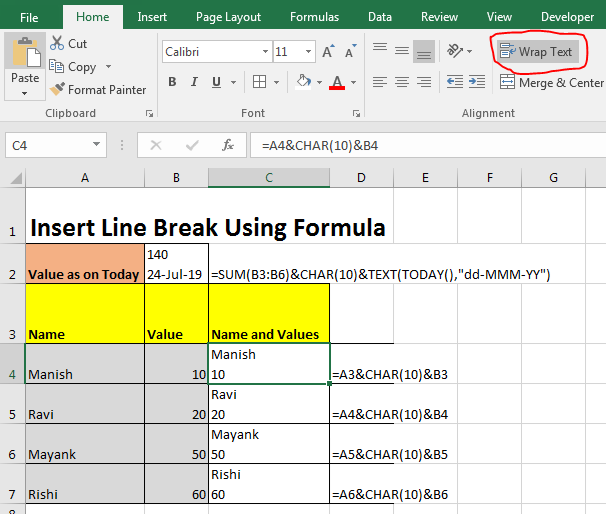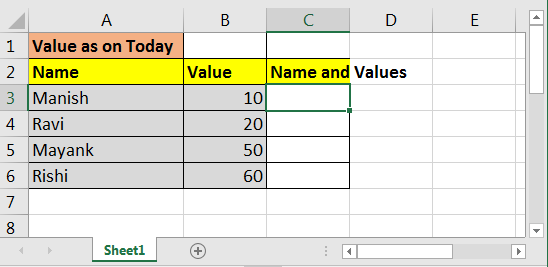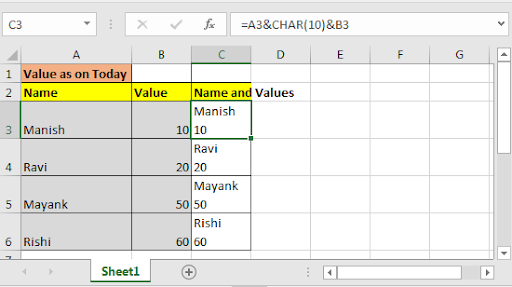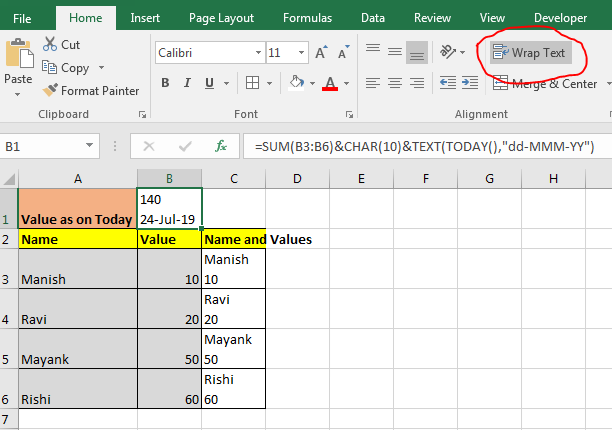# How to Insert a Line Break using Formula in Excel

So, normally we use ALT+ENTER key combination to add a line break in excel within a cell. This command wraps the text and adds an excel line break each time this key combination pressed. How do we do the same thing using formula? How do we insert line break in excel using formula? Let’s find out…Generic Formula to Insert Excel Line Break

=”text/formula”&CHAR(10)&”text/formula”

*Important note: The cell should be wrapped.

• Here ”text/formula” can be any text or formula.
• &CHAR(10)& this is equivalent to ALT+ENTER.
• & is concatenation operator in excel. It adds any two strings.
• CHAR function returns a character when you supply a valid character code. Character code for line break is 10 in Microsoft. For mac users, it's 13.

Excel Line Break Formula Example

So, here we have this data.In adjacent cells of values, we need name along with values. Values should be with a line break.
Write this formula in the cell C3 and click on Wrap Text on Home Tab. if it's already not clicked. And drag it down to cell C6.

=A3&CHAR(10)&B3

And this now how it looks like.See how we added value below with a line break.
Let's take another example, in cell B1 I want to sum values, and in the same cell, i want to show today's date. Date should be entered with a line break.
So to do this we can write this formula to add line breaks.

=SUM(B3:B6)&CHAR(10)&TEXT(TODAY(),"dd-MMM-YY")So yeah guys, you can enter a new line in excel (or say a line break in excel) using CHAR function of excel and concatenation operator (&). You just need to know enable the Wrap Text for cell. Line break in code in Excel is 10 for windows and 13 for Mac.

Related Articles:

How to use New Line In Cell in Excel

How to Count the number of newlines in a cell

Popular Articles:

50 Excel Shortcuts to Increase Your Productivity

How to use the VLOOKUP Function in Excel

How to use the COUNTIF function in Excel

How to use the SUMIF Function in Excel

Terms and Conditions of use

The applications/code on this site are distributed as is and without warranties or liability. In no event shall the owner of the copyrights, or the authors of the applications/code be liable for any loss of profit, any problems or any damage resulting from the use or evaluation of the applications/code.